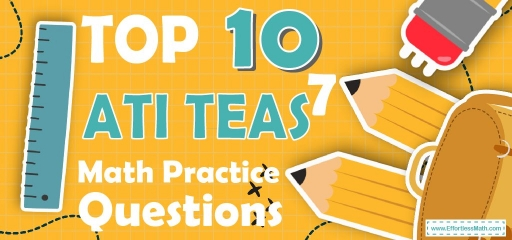# Top 10 ATI TEAS 7 Math Practice QuestionsTaking the ATI TEAS 7 Math test in a few weeks? The best way to prepare for your ATI TEAS Math test is to work through as many ATI TEAS Math practice questions as possible. Here are the top 10 ATI TEAS Math practice questions to help you review the most important ATI TEAS Math concepts. These ATI TEAS Math practice questions are designed to cover mathematics concepts and topics that are found on the actual test. The questions have been fully updated to reflect the latest 2022 ATI TEAS guidelines. Answers and full explanations are provided at the end of the post.

Start your ATI TEAS Math test prep journey right now with these sample ATI TEAS Math questions.

## ATI TEAS Math Practice Questions

1- If $$(4.2 + 4.3 + 4.5) x = x$$, then what is the value of $$x$$?

A. 0

B. $$\frac{1}{10}$$

C. 1

D. 10

2- The sum of two numbers is $$x$$. If one of the numbers is 9, then two times the other number would be what?

A. $$2X$$

B. $$2 + x × 2$$

C. $$2(x + 9)$$

D. $$2(x -9)$$

3- A circle has a diameter of 8 inches. What is its approximate circumference?

A. 6.28

B. 25.12

C. 34.85

D. 35.12

4- If $$x = \frac{5}{7}$$ then $$\frac{1}{x}=$$ ?

A. $$\frac{7}{5}$$

B. $$\frac{5}{7}$$

C. 5

D. 7

5- If $$6.5 < x ≤ 9.0$$, then $$x$$ cannot be equal to:

A. 6.5

B. 9

C. 7.2

D. 3.5

6- Which of the following is the product of $$1\frac{1}{2}$$ and $$4\frac{3}{5}$$?

A.$$9\frac{6}{10}$$

B. $$6\frac{9}{10}$$

C. $$10\frac{6}{9}$$

D. $$4\frac{2}{3}$$

7- How many $$\frac{1}{4}$$ pound paperback books together weigh 30 pounds?

A. 80

B. 95

C. 105

D. 120

8- $$\frac{7}{25}$$ is equals to:

A. 0.30

B. 2.80

C. 0.03

D. 0.28

9- In the simplest form, $$\frac{18}{24}$$ is:

A. $$\frac{2}{3}$$

B. $$\frac{3}{2}$$

C. $$\frac{4}{3}$$

D. $$\frac{3}{4}$$

10- The sum of 8 numbers is greater than 240 and less than 320. Which of the following could be the average (arithmetic mean) of the numbers?

A. 30

B. 35

C. 40

D. 45

## Best ATI TEAS Math Prep Resource for 2022

1- A
$$(4.2 + 4.3 + 4.5) x = x$$
$$13x = x$$
Then $$x = 0$$

2- D
$$a + b = x$$
$$a = 9→9 + b = x → b = x – 9$$
$$2b = 2(x – 9)$$

3- B
$$C = 2πr$$
$$C = 2π × 4 = 8π$$
$$π = 3.14 → C = 8π = 25.12$$

4- A
$$\frac{1}{x}=\frac{\frac{1}{1}}{\frac{5}{7}}=\frac{7}{5}$$

5- D
If $$6.5 < x ≤ 9.0$$, then $$x$$ cannot be equal to: $$3.5$$

6- B
Converting mixed numbers to fractions, our initial equation becomes
$$\frac{3}{2} × \frac{23}{5}$$
Applying the fractions formula for multiplication
$$\frac{(3 × 23)}{(2 × 5) }=\frac{69}{10}=6\frac{9}{10}$$

7- D
$$30 \div \frac{1}{4}=30 \times 4=120$$

8- D
$$\frac{7}{25} = 0.28$$

9- D
$$\frac{18}{24}=\frac{3}{4}$$

10- B
$$\frac{240}{8} < x < \frac{320}{8}$$
$$30 < x < 40$$
THEN:
35 is correct

Looking for the best resource to help you succeed on the ATI TEAS Math test?

## The Best Books to Ace the ATI TEAS Math Test

### What people say about "Top 10 ATI TEAS 7 Math Practice Questions - Effortless Math: We Help Students Learn to LOVE Mathematics"?

No one replied yet.

X
52% OFF

Limited time only!

Save Over 52%

SAVE $40 It was$76.99 now it is \$36.99